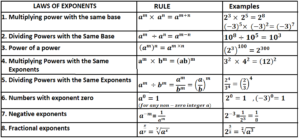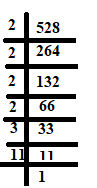October 7, 2022

# Laws of exponents class 7

Laws of exponents class 7

Exponents are used to express large numbers in shorter form to make them easy to read, understand, compare and operate upon. The base is the number that is being repeated as a factor in the multiplication. For example,

The exponent tells you how many times the base is repeated as a factor in the multiplication . Here exponent is 3 .

Contents

# Laws of exponents  with examples class 7## Laws of exponents Class 7 questions

Laws of exponents class 7 (solved examples)

Simplify the following using laws of exponents class 7

Example 1. Find the value of  .

Solution.  Since for any non- zero integer , so    = (1-1)  x 1  = 0 x 1 =0.

Hence = 0 .

Example 2 . Evaluate

Solution. Using the rule , we get   =

=

=0

Example 3. Express 528 in exponential notation.

Solution.  528= 2 x 2 x 2 x 2 x 3 x 11

=Example 4. Find x such that .

Solution. Using the law of exponents  , we get

On both the sides , powers have the same base , so their exponents must be equal. Therefore 4x= 20  or x=5.

Hence the value of x is 5.

Example 5. Solve   .

Solution. = =        ( Using the rule )

=

Example 6. Find the value of  if,

Solution.

Example 7. By what number should we multiply so that the product may be equal to ?

Solution. Let be multiplied by so that the product may be equal to .
According to question,

or

=256                    ( Using the rule )

Therefore , should be multiplied by 256 so that the product is equal to .

Example 8. Solve

Solution.  =     =

Example 9. Find m,  so that  .

Solution.  We have,

( Using the law of exponents  )

On comparing both the sides, we get 9= 2m-1

. Hence

Example 10.  If ÷ , then find the value of

Solution. For any non- zero integer .Hence   ÷ 1

On cubing both the sides,  we get .

### Powers and Exponents worksheet pdf

#### Exponents and Powers Class 7 Extra Questions

A. Fill in the blanks:

(i) ……….

(ii)

(iii)   =………

(iv)   =…………..

(v) For any two non-zero integers any ÷   is equal to……………..

(vi)  ( ÷ =……….

(vii) = …………….

(viii)   =……………..

(ix)  The prime factorisation of 216 in exponential form is = ………………

(x) 2401 as a power of 7 = ……………..

B. State whether the following statements are  True /False.

(i)

(ii)

(iii) For a non-zero rational number ÷

(iv)

(v)

(vi) ÷   is true for all non-zero values of .

(vii) 1° x 01 =1

(viii)

(ix)

(x) xm + xm = x2m, where x is a non-zero rational number and m is a positive integer.

C. (i) By what number should we multiply so that the product may be equal to ?

(ii) Find so that

(iii) If a=2 and b=3 then find the value of .

(iv) If ÷  = then find the value of .

(v) For non-zero numbers  and  , ÷ , where , is equal to ……………….

(vi) Express each of the following in single exponential form,
(a) 33 x 43
(b) 24 x 42
(c) 62 x 82
(d) (- 6)5 x (- 6)
(e) (- 3)3 x (- 10)3
(f) (- 11)2 x (- 5)2

Ans. A. (i) 1        (ii) -1      (iii)        (iv)      (v)     (vi)       (vii) -1     (viii) 8       (ix)       (x)

B. (i) False     (ii) True      (iii) True      (iv)  False    (v) True     (vi) True    (vii) False    (viii) True  (ix) False    (x) False

C. (i)  27    (ii) x=8    (iii)  17     (iv) 3      (v)     (vi) a)      b)     c)     d)    e)   f)

You must be also interested in:#### Bina singh

View all posts by Bina singh →
List of Google Scholarships IBPS Clerk 2022 Mains Exam Admit card 2022 CUET-PG results Scheduled Exams in October 2022 SBI Notification for the 1673 Probationary Officers